### Home > CALC > Chapter 5 > Lesson 5.3.2 > Problem5-123

5-123.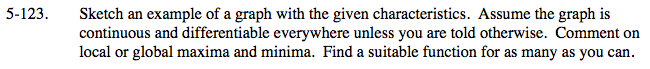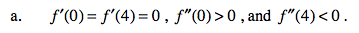The 2nd Derivative Test states that if:
If f ′(a) = 0 AND f ′′(a) > 0, then x = a is the location of a local minimum on f(x).
If f ′(a) = 0 AND f ′′(a) < 0, then x = a is the location of a local maximum on f(x).
But if f ′(a) = 0 AND f ′′(a) = 0, then the test is inconclusive...f(a) might be a max, min or inflection point.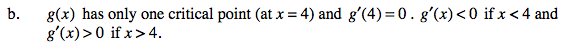The 1st Derivative Test states that if:
If f '(a) = 0 AND f ′(x) changes from negative to positive values at x = a, then x = a is the location of a local minimum on f(x).
If f ′(a) = 0 AND f ′(x) changes from positive to negative values at x = a, then x = a is the location of a local maximum on f(x).
But if f ′(a) = 0 AND f ′(x) does not change signs at x = a, then x = a is the location of an inflection point on f(x).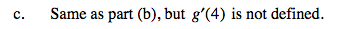Functions can have an undefined slope (derivative) at a cusp, endpoint, jump, hole or vertical tangent.
Do any of those scenarios match the given one: g ′(4) = DNE but g ′(x) changes from negative to positive at x = 4.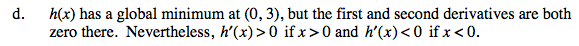Refer to hint in part (b), the 1st Derivative Test.